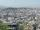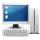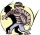# Profit growth

The profit of a company increased by 25% during the year 1992, increased by 40% during the year 1993, decreased by 20% in the year 1994 and increased by 10% during the year 1995. Find the average growth in the profit level over the four years periods?

Result

p =  11.399 %

#### Solution:Leave us a comment of example and its solution (i.e. if it is still somewhat unclear...):

Showing 0 comments:Be the first to comment!#### To solve this example are needed these knowledge from mathematics:

Looking for a statistical calculator? Need help calculate sum, simplify or multiply fractions? Try our fraction calculator. Our percentage calculator will help you quickly calculate various typical tasks with percentages.

## Next similar examples:

1. The cityAt the end of 2010 the city had 248000 residents. The population increased by 2.5% each year. What is the population at the end of 2013?
2. Investment1000\$ is invested at 10% compound interest. What factor is the capital multiplied by each year? How much will be there after n=12 years?
3. ComputerThe computer was purchased 10000,-. Each year, the price of a computer depreciates always the same percentage of the previous year. After four years, the value of the computer is reduced to 1300,- How many percent was depreciated price of the computer each
4. The crimeThe crime rate of a certain city is increasing by exactly 7% each year. If there were 600 crimes in the year 1990 and the crime rate remains constant each year, determine the approximate number of crimes in the year 2025.
5. The city 2Today lives 167000 citizens in city. How many citizens can we expect in 11 years if their annual increase is 1%?
6. Radioactive materialA radioactive material loses 10% of its mass each year. What proportion will be left there after n=6 years?
7. Account operationsMy savings of php 90,000 in a bank earns 6% interest in a year. If i will deposit additional php 10,000 at the end of 6 months, how much money will be left if i withdraw php 25,000 after a year?
8. Five membersWrite first 5 members geometric sequence and determine whether it is increasing or decreasing: a1 = 3 q = -2
9. Tenth memberCalculate the tenth member of geometric sequence when given: a1=1/2 and q=2
10. Geometric progression 2There is geometric sequence with a1=5.7 and quotient q=-2.5. Calculate a17.
11. A perineumA perineum string is 10% shorter than its original string. The first string is 24, what is the 9th string or term?
12. GP - 8 itemsDetermine the first eight members of a geometric progression if a9=512, q=2
13. Geometric progression 48,4√2,4,2√2
14. Six termsFind the first six terms of the sequence a1 = -3, an = 2 * an-1
15. Geometric sequence 4It is given geometric sequence a3 = 7 and a12 = 3. Calculate s23 (= sum of the first 23 members of the sequence).
16. Theorem proveWe want to prove the sentense: If the natural number n is divisible by six, then n is divisible by three. From what assumption we started?
17. CalculationHow much is sum of square root of six and the square root of 225?